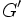# Group of nilpotency class two whose derived subgroup is 2-divisible

This article defines a group property: a property that can be evaluated to true/false for any given group, invariant under isomorphism
View a complete list of group properties
VIEW RELATED: Group property implications | Group property non-implications |Group metaproperty satisfactions | Group metaproperty dissatisfactions | Group property satisfactions | Group property dissatisfactions

## Definition

A group$G$ is termed a group of nilpotency class two whose derived subgroup is 2-divisible if it satisfies both the following conditions:

1.$G$ is a group of nilpotency class two.
2. The derived subgroup$G'$ is a 2-divisible group, i.e., every element of the derived subgroup has a square root in the derived subgroup.

## Relation with other properties

### Stronger properties

Property Meaning Proof of implication Proof of strictness (reverse implication failure) Intermediate notions